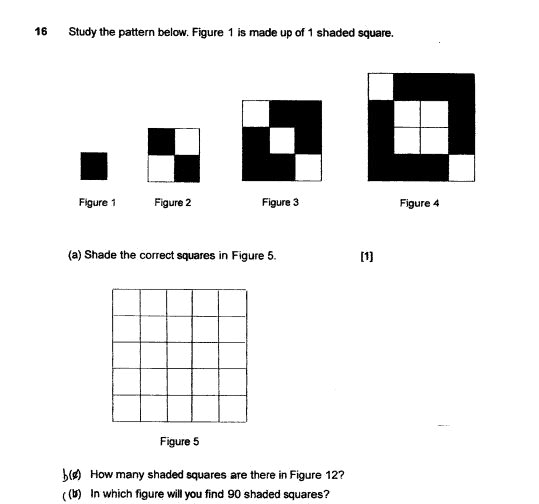# QuestionPlease help. Thank you.

Source: Ai Tong

2 Answers

# Answer

a)
Shade the squares indicated as ‘(X)’ with ‘X’ as unshaded :
.X (X)(X)(X)(X)
(X) X X X (X)
(X) X X X (X)
(X) X X X (X)
(X)(X)(X)(X) X

(b)
shaded squares : (Fig no. – 2 ) x 4 + 2
(12 – 2) x 4 + 2 = 42
unshaded squares : total squares – shaded squares
total square = Fig no. x Fig no.
Fig no. : shaded squares : unshaded squares : total squares
Fig 1 : 1 : 0 : 1
Fig 2 : 2 : 2 : 4
Fig 3 : 6 : 3 : 9
Fig 4 : 10 : 6 : 16

Fig 12 : 42 : 102 : 144

(c)
90 – 2 = 88
88/4 = 22

Ans : (a) as above; (b) 42 shaded squares; (c) Figure 22.

0 Replies 0 Likes ✔Accepted Answer